Here we are providing Class 12 Maths Important Extra Questions and Answers Chapter 2 Inverse Trigonometric Functions. Class 12 Maths Important Questions are the best resource for students which helps in Class 12 board exams.

## Class 12 Maths Chapter 2 Important Extra Questions Inverse Trigonometric Functions

### Inverse Trigonometric Functions Important Extra Questions Very Short Answer Type

Question 1.
Find the principal value of sin-1 ( $$\frac { 1 }{ 2 }$$ )
Solution:
$$\sin ^{-1}\left(\frac{1}{2}\right)=\frac{\pi}{6} \in\left[-\frac{\pi}{2}, \frac{\pi}{2}\right]$$
Hence, the principal value of sin-1 ( $$\frac { 1 }{ 2 }$$ ) is $$\frac{\pi}{6}$$

Question 2.
What is the principal value of:
cos-1 (cos $$\frac{2 \pi}{3}$$ + sin-1 (sin $$\frac{2 \pi}{3}$$ ) ?
Solution:Question 3.
Find the principal value of:
tan-1 (√3)- sec-1 (-2). (A.I.C.B.S.E. 2012)
Solution: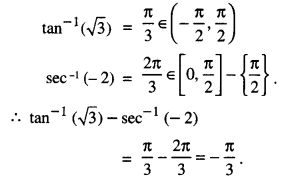Question 4.
Evaluate : tan -1 ( 2 cos (2 sin-1 ( $$\frac{1}{2}$$ )))
Solution: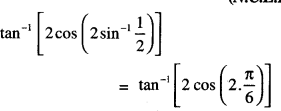Question 5.
Find the value of tan-1(√3) – cot-1(-√3). (C.B.S.E. 2018)
Solution:
tan-1(√3) – cot-1(—√3)
$$=\frac{\pi}{3}-\left(\pi-\frac{\pi}{6}\right)=-\frac{\pi}{2}$$

Question 6.
If sin-1 ( $$\frac { 1 }{ 3 }$$ ) + cos-1 x = $$\frac{\pi}{2}$$, then find x.(C.B.S.E. 2010C)
Solution:
sin-1 ( $$\frac { 1 }{ 3 }$$ ) + cos-1 x = $$\frac{\pi}{2}$$
⇒ x = 1/3
[sin-1 x + cos-1 x = $$\frac{\pi}{2}$$

Question 7.
If sec-1 (2) + cosec-1 (y) = $$\frac{\pi}{2}$$ , then find y.
Solution:
sec-1 (2) + cosec-1 (y) = $$\frac{\pi}{2}$$
⇒ y = 2 [∵ sec-1 x + cosec-1 x= $$\frac{\pi}{2}$$ ]

Question 8.
Write the value of sin [ $$\frac{\pi}{3}$$ – sin -1 ( $$\frac { -1 }{ 2 }$$ ) ]
Solution:
sin [ $$\frac{\pi}{3}$$ – sin-1 ( $$\frac { -1 }{ 2 }$$ ) ]
= sin [ $$\frac{\pi}{3}$$ + sin-1 ( $$\frac { 1 }{ 2 }$$ ) ]
[∵ sin-1 (-x) = -sin-1x]
= sin ( $$\frac{\pi}{3}+\frac{\pi}{6}$$ ) = sin $$\frac{\pi}{2}$$ = 1

Question 9.
Prove the following: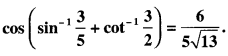Solution: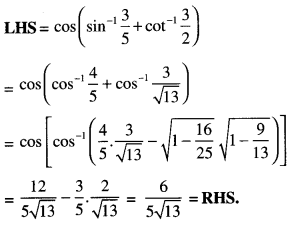Question 10.
If tan-1 x + tan-1y = $$\frac{\pi}{4}$$ , xy < 1, then write the value of the x + y + xy (A.I.C.B.S.E. 2014)
Solution:
We have tan-1 x + tan-1y = $$\frac{\pi}{4}$$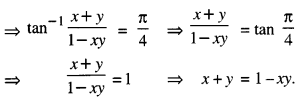Hence x + y + xy = 1.

Question 11.
Prove that: 3 sin-1 x = sin-1(3x – 4x2);
x ∈ [$$\frac { -1 }{ 2 }$$ , $$\frac { 1 }{ 2 }$$] (C.B.S.E 2018)
Solution:
To prove: 3 sin-1 x = sin-1(3x – 4x2)
Put sin-1 x = θ
so that x = sin θ.
RHS = sin-1 (3 sin θ – 4 sin3 θ)
= sin-1 (sin 3θ) = 3θ = 3 sin-1x = LHS.

### Inverse Trigonometric Functions Important Extra Questions Short Answer Type

Question 1.
Express sin-1 ( $$\frac{\sin x+\cos x}{\sqrt{2}}$$ )
Where $$-\frac{\pi}{4}$$ < x < $$\frac{\pi}{4}$$, in the simples form.
Solution:Question 2.
Prove that :
$$\cos ^{-1} \frac{12}{13}+\sin ^{-1} \frac{3}{5}=\sin ^{-1} \frac{56}{65}$$   (A.I.C.B.S.E. 2019; C.B.S.E. 2010)
Solution:Question 3.
Prove that :
sin -1 $$\frac{8}{17}$$ + cos -1 $$\frac{4}{5}$$ = cos-1 $$\frac{36}{77}$$ (A.I.C.B.S.E. 2019)
Solution:
L.H.S = sin-1 $$\frac{8}{17}$$ + cos-1 $$\frac{4}{5}$$
= tan-1 $$\frac{8}{15}$$ + tan-1$$\frac{3}{4}$$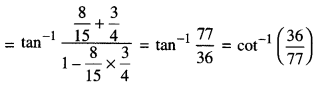= R.H.S

Question 4.
Solve the following equation:
$$\tan ^{-1}\left(\frac{x+1}{x-1}\right)+\tan ^{-1}\left(\frac{x-1}{x}\right)=\tan ^{-1}(-7)$$ (A.I.C.B.S.E. 2019; C)
Solution: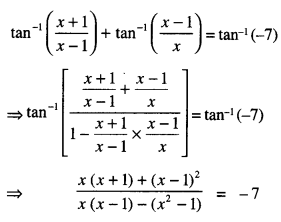⇒ 2x2 – 8x + 8 = 0
⇒ x2 – 4x + 4 = 0
⇒ (x – 2)2 = 0.
Hence, x = 2.

Question 5.
Solve the following equation:
2 tan-1(sin x) = tan-1 (2 sec x), x ≠ $$\frac{\pi}{2}$$   (C.B.S.E. (F) 2012)
Solution:
2 tan-1(sinx) = tan-1 (2 secx)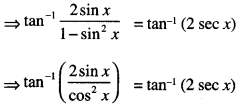⇒ tan -1 (2sec x tan x) = tan-1 (2sec x)
⇒ 2 sec x tan x = 2 sec x
tan x = 1 [∵ sec x ≠ 0 ]
Hence, x= $$\frac{\pi}{2}$$

Question 6.
Solve the following equation
cos (tan-1 x) = sin ( cot -1$$\frac { 3 }{ 4 }$$ )
(A.I.C.B.S.E. 2013)
Solution:
We have:
cos (tan-1 x) = sin ( cot -1$$\frac { 3 }{ 4 }$$ )
cos (tan-1 x) = sin ( sin -1$$\frac { 4 }{ 5 }$$ )
cos (tan-1 x) = $$\frac { 4 }{ 5 }$$
tan-1 x = cos-1$$\frac { 4 }{ 5 }$$
⇒ tan-1 x = tan -1$$\frac { 3 }{ 4 }$$
Hence x = $$\frac { 3 }{ 4 }$$

Question 7.
Prove that
3cos-1 x = cos-1 (4x3 – 3x), x ∈ [ $$\frac { 1 }{ 2 }$$ , 1 ]
Solution:
Put x = cos θ in RHS
As 1/2 ≤ x ≤ 1
RHS = cos-1 (4cos3 θ – 3cos θ),
= cos-1 (cos 3θ) = 3θ = 3cos-1 x = L.H.S

### Inverse Trigonometric Functions Important Extra Questions Long Answer Type 1

Question 1.
prove that $$\frac { 1 }{ 2 }$$ ≤ x ≤ 1, then
$$\cos ^{-1} x+\cos ^{-1}\left[\frac{x}{2}+\frac{\sqrt{3}-3 x^{2}}{2}\right]=\frac{\pi}{3}$$ (CBSE. Sample paper 2017 – 18)
Solution: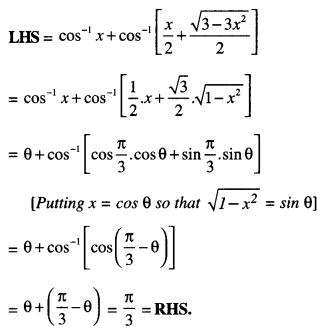Question 2.
Find the value of :
$$\tan ^{-1}\left(\frac{x}{y}\right)-\tan ^{-1}\left(\frac{x-y}{x+y}\right)$$
Solution: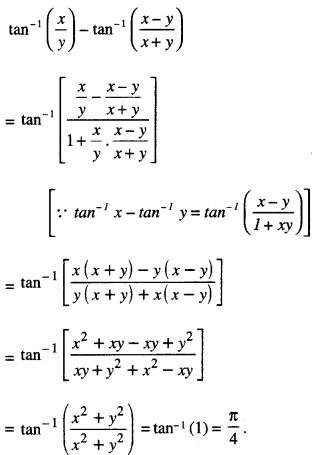Question 3.
Prove that :
tan -1( $$\frac{1}{2}$$ ) + tan -1 ( $$\frac{1}{5}$$ ) + tan -1( $$\frac{1}{8}$$ ) = $$\frac{\pi}{4}$$ (C.B.S.E. 2013: A.I.C.B.S.E. 2011)
Solution: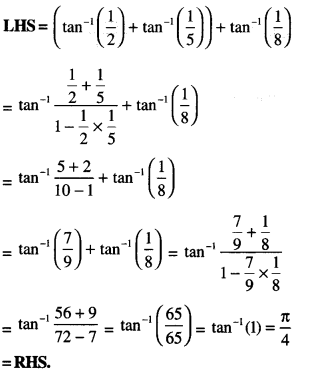Question 4.Solution: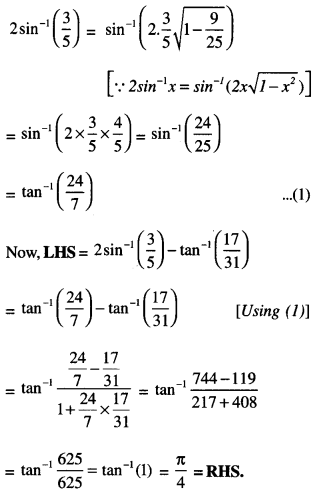Question 5.Solution:
Put cos -1 (a/b) = θ so that cos θ = a/b.Question 6.Solution: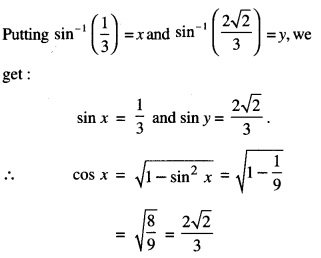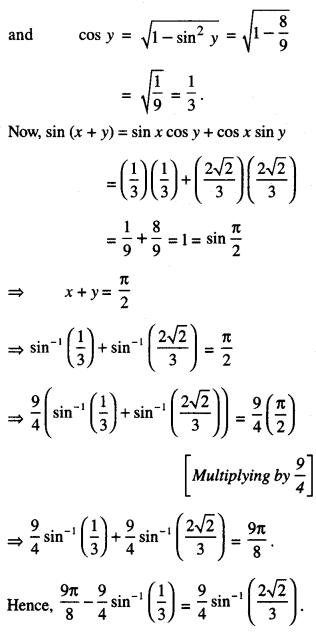Question 7.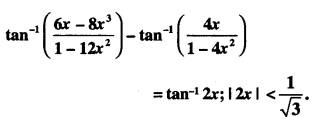( A.I.C.B.S.E. 2016)
Solution: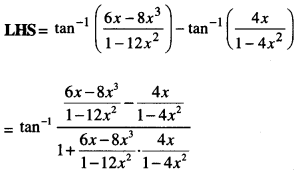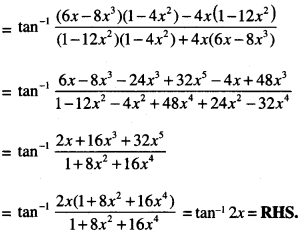Question 8.
$$\tan ^{-1} \frac{1}{5}+\tan ^{-1} \frac{1}{7}+\tan ^{-1} \frac{1}{3}+\tan ^{-1} \frac{1}{8}=\frac{\pi}{4}$$ (C.B.S.E. 2016)
Solution:Question 9.
Solve for x : 2 tan-1 (cos x ) = tan-1(2 cosec x). (C.B.S.E. 2016)
Solution:
2 tan-1 (cos x ) = tan-1 (cos x) + tan-1 (cos x)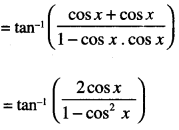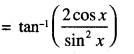= tan-1 (2cot x cosec x) …(1)
Now 2 tan-1(cos x) = tan-1(2 cosec x)
⇒ tan-1(2 cot x cosec x) = tan-1 (2cosec x)
[Using (1)]
⇒ 2 cot x cosec x = 2 cosec x
⇒ cotx cosecx = cosec x
⇒  sin x = tan x sin x
⇒either sin x = 0 or tan x = 1.
Hence, x = nπ ∀ n ∈ Z or x
= πm + $$\frac{\pi}{4}$$ ∀ m ∈ Z

Question 10.
If tan-1 $$\left(\frac{x-2}{x-4}\right)$$ + tan-1 $$\left(\frac{x+2}{x+4}\right)$$ = $$\frac{\pi}{4}$$ find the value of ‘x’   (A.I.C.B.S.E. 2014)
Solution:
We have⇒ 2x2 – 16 = -12
⇒ 2x2 = 16 – 12
⇒ 2x2 = 4
⇒ x2 = 2
Hence x = ±√2

Question 11.
If tan-1 $$\left(\frac{x-3}{x-4}\right)$$ + tan-1 $$\left(\frac{x+3}{x+4}\right)$$ = $$\frac{\pi}{4}$$ find the value of ‘x’ (A.I.C.B.S.E. 2017)
Solution: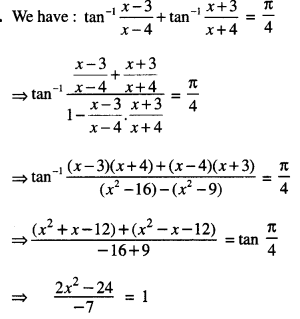⇒ 2x2 – 24 = -7
⇒ 2x2 = 17
⇒ 2x2 = 4
⇒ x2 = 17/2
Hence x = $$\pm \sqrt{\frac{17}{2}}$$

Question 12.
Prove that : $$\tan ^{-1} \sqrt{x}=\frac{1}{2} \cos ^{-1}\left(\frac{1-x}{1+x}\right)$$
x∈ [0,1] (C.B.S.E. 2019C)
Solution:
Let tan -1 √x = θ
So that √x = tan θ i.e. x = tan2θ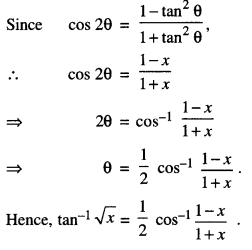Question 13.
Solve for x : tan -1 (2x) + tan -1 (3x) = $$\frac{\pi}{4}$$ (C.B.S.E. 2019)
Solution:
The given equation is
tan -1 (2x) + tan -1 (3x) = $$\frac{\pi}{4}$$ …. (1)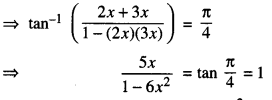⇒ 5x = 1 – 6x2
⇒ 6x2 + 5x – 1 = 0
⇒ x = -1 or x = $$\frac{1}{6}$$
Hence, x = $$\frac{1}{6}$$
[∵ x = -1 does not satisfy (1)]

Question 14.
Solve : tan-1 4x + tan-1 6x = $$\frac{\pi}{4}$$ (C.B.S.E. 2019)
Solution:
We have tan-1 4x + tan-1 6x = $$\frac{\pi}{4}$$⇒ 10x = 1 – 24x2
⇒ 24x2 + 10x – 1 = 0
⇒ 24x2+ 12x – 2x – 1 = 0
⇒ 12x(2x + 1) – 1 (2x + 1) = 0
⇒ (2x+1)(12x – 1) = 0
⇒ 2x + 1 = 0 or 12x – 1 = 0
x = $$-\frac{1}{2}$$ or x = $$\frac{1}{2}$$.
Hence, x = $$-\frac{1}{2}$$ or $$\frac{1}{12}$$
As x = $$-\frac{1}{2}$$ does not satisfy the given equation.
Hence x = $$-\frac{1}{12}$$

Question 15.
If (tan-1 x )2 + (cot-1 x )2 = $$\frac{5 \pi^{2}}{8}$$ , then find ‘x’ (C.B.S.E. 2015)
Solution:
We have
(tan-1 x )2 + (cot-1 x )2 = $$\frac{5 \pi^{2}}{8}$$
Put tan-1 x = t so that cot-1x = $$\frac{\pi}{2}$$ – t
∴ (1) become : t2 + ($$\frac{\pi}{2}$$ – t)2 = $$\frac{5 \pi^{2}}{8}$$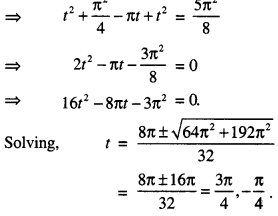When tan-1x = $$\frac{3 \pi}{4}$$
then x = tan $$\frac{3 \pi}{4}$$ = -1.
When tan-1 x = $$-\frac{\pi}{4}$$,
then x = tan($$-\frac{\pi}{4}$$) = -1.
Hence, x = -1.

Question 16.
Write : tan -1$$\frac{1}{\sqrt{x^{2}-1}}$$ , |x| > 1 in the simplest form.
Solution:
Put x = sec θQuestion 17.
Prove that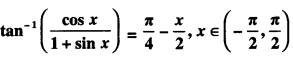(C.B.S.E. 2012)
Solution: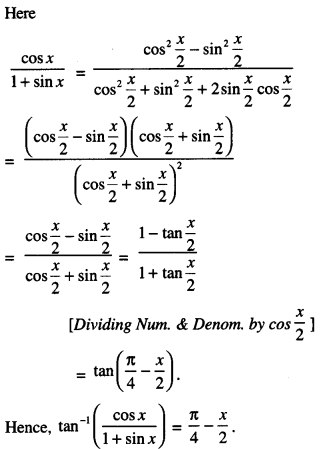Question 18.
Prove that :
tan-1x + tan-1 $$\frac{2 x}{1-x^{2}}$$ = tan -1$$\frac{3 x-x^{3}}{1-3 x^{2}}$$ (N.C.E.R.T
Solution:
Put x = tan θ so that θ = tan -1 x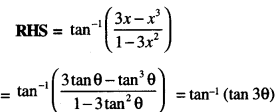= 3θ = 3 tan-1x = tan-1x + 2tan-1x
= tan-1x + tan-1$$\frac{2 x}{1-x^{2}}$$
= L.H.S.

Question 19.
Simplify : tan-1 [ $$\left[\frac{a \cos x-b \sin x}{b \cos x+a \sin x}\right]$$]
If $$\frac{a}{b}$$ tan x > -1    (N.C.E.R.T)
Solution: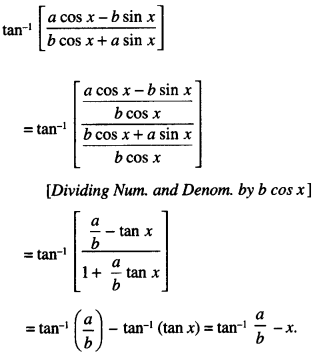Question 20.
Prove that tan -1 [ $$\frac{\sqrt{1+x}+\sqrt{1-x}}{\sqrt{1+x}-\sqrt{1-x}}$$ ]
= $$\frac{\pi}{4}+\frac{1}{2}$$ cos-1 x ; $$-\frac{1}{\sqrt{2}}$$ ≤ x ≤ 1. (C.B.S.E. 2019C)
Solution: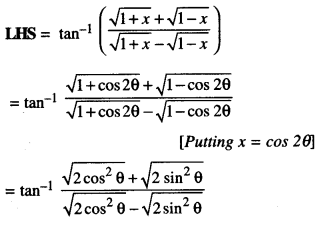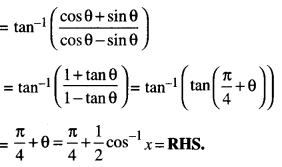Question 21.
Show that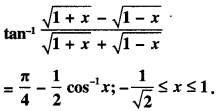(N.C.E.R.T.A.I. CBSE 2014)
Solution: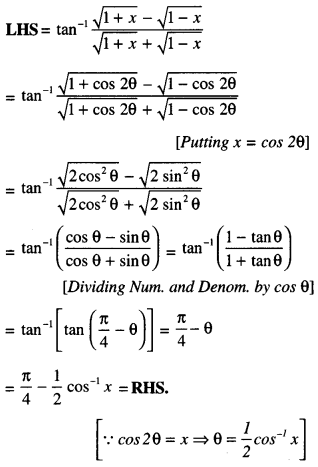Question 22.
cos[tan-1{sin (cot-1x)}] = $$\sqrt{\frac{1+x^{2}}{2+x^{2}}}$$
(A.I. C.B.S.E. 2010)
Solution:
Put cot-1 = θ so that x = cot θ
∴ sin θ = $$\frac{1}{\sqrt{1+x^{2}}}$$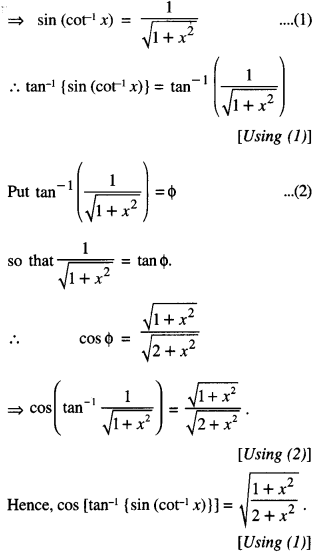Question 23.
Find the value of sin (2 tan-1 1/4) + cos (tan-1 2√2)   (C.B.S.E. Sample Paper 2018 – 2019)
Solution:
Put tan-1 1/4 = θ so that θ = 1/4
Now, sin 2θ = $$\frac{2 \tan \theta}{1+\tan ^{2} \theta}$$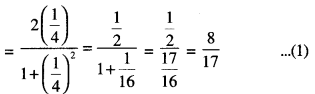To evaluate cos(tan-1 2√2) :
Put tan-12√2 = Φ
So that tan Φ = 2√2
cos Φ = 1/3
Hence, sin (2tan-1(1/4)) + cos (tan-12√2)
= sin 2θ + cos Φ = $$\frac{8}{17}+\frac{1}{3}=\frac{41}{51}$$
[Using (1) and (2)]

Question 24.
Solve for x:
tan-1(x – 1) + tan-1x + tan-1(x + 1) = tan-1(3x).    (A.I.C.B.S.E. 2016)
The given equation is:
tan-1(x —1) + tan-1(x) + tan-1(x + 1) = tan-13x
tan-1(x— 1)+tan-1(x+ 1) = tan-13x – tan-1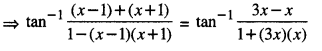⇒ 1 + 3x2 = 2 – x2
⇒ 4x2 = 1
⇒ x2 = 1/4
⇒ x = $$\pm \frac{1}{2}$$
Hence, x = 0, $$\pm \frac{1}{2}$$